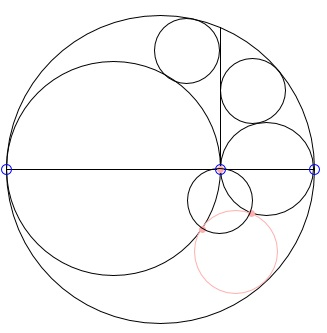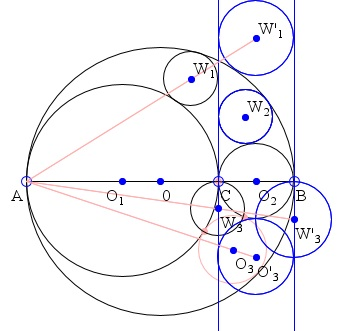### This applet requires Sun's Java VM 2 which your browser may perceive as a popup. Which it is not. If you want to see the applet work, visit Sun's website at https://www.java.com/en/download/index.jsp, download and install Java VM and enjoy the applet.

 What if applet does not run?

ExplanationOne of the properties of the arbelos noticed and proved by Archimedes in his Book of Lemmas is that the two small circles inscribed into two pieces of the arbelos cut off by the line perpendicular to the base through the common point of the two small semicircles are equal. The circles have been known as Archimedes' Twin Circles. More than 2200 years after Archimedes, L. Bankoff (1974) has found another circle equal to the twins.The best way to see the circle is to reflect the arbelos in the common base of its constituent semicircles. Proposition 6 of the Book of Lemmas deals with the circle tangent to all three semicircles. The two points of tangency with the small semicircles together with the point C in the applet form a triangle whose circumcenter has the same radius as Archimedes' twins!For definiteness' sake, let the semicircles have centers O, O1, O2. Following Bankoff's convention, a circle will be denoted by the same letter as its center enclosed in a couple of parentheses. Thus, e.g. the big semicircle is the top part of (O). The twin circles are, say, (W1) and (W2), and the circle due to Proposition 6 is (O3). The circle in question is (W3).

To prove this result we shall use the inversion with center A and the power equal to AC·AB.

The inversion swaps B and C, and further

 The circle (O1) becomes the line perpendicular to AB at B. The circle (O) becomes the line perpendicular to AB at C. The circles (O2) and (W2) are self-inverse. The circle (W1) inverts into (W'1), tangent to lines the two vertical lines and the circle (O) externally. The circle (O3) inverts into (O'3), tangent to the vertical lines and to the self-inverse (O2). The circle (W3) becomes (W'3), tangent to AB at B and orthogonal to (O2) and (O'3.

Denote the radii of the circles (O), (O1), (O2), (O3),(W1), (W2), (W3), (O'1), (O'3), (W'1), (W'3) as R, R1, R2, R3, p1 = p2 = p, p3, R'1, R'3, p', p'3, respectively. Note that p' = R2.

We have the following:

1. Since A is the external center of similitude of (W1) and (W'1),

 p/p' = (2R1 - p)/(2R1 + R2),

from which

 p = R1·R2/R = R1·R2/(R1 + R2).

2. In the triangles ACW3 and ABW'3

 p3/p'3 = AC/AB = R1/R

and it follows that

 p3 = R1·p'3/R = R1·R2/R.

Note that in terms of R1 and R2, Archimedes' formula becomes

 R3 = R·R1·R2/(R12 + R1·R2 + R22).

### Remark

As an aside, the foregoing reminds me of another question. Two brothers that were born virtually at the same time on the same day in the same year to the same parents are not twins. How can this be?

### References

1. L. Bankoff, Are the Twin Circles of Archimedes Really Twin, Mathematics Magazine, Vol. 47, No. 4 (Sept., 1974), 214-218### Inversion - Introduction